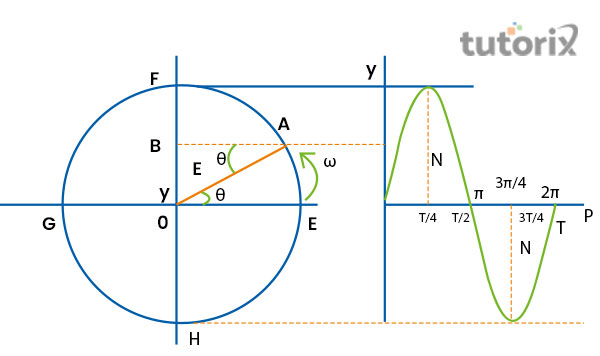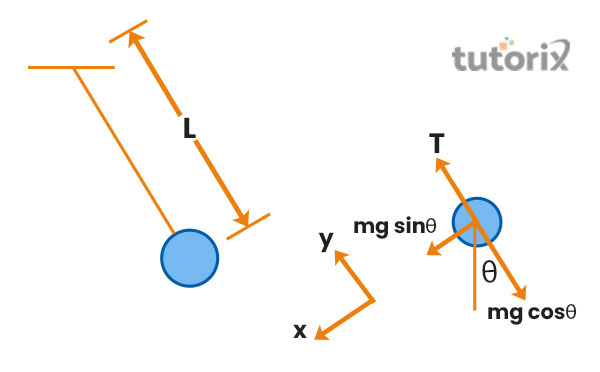# Simple harmonic motion and uniform circular motion

## Introduction

Simple harmonic motion can be defined as a periodic motion of a particular point in a straight line like its acceleration is to the fixed point of that line and its acceleration is directly proportional to the distance of it from a particular point. On the other hand, Uniform Circular Motion denotes the motion of a particle that is moving at a perpetual speed along the circular path. In this case, the speed of the particular component remains constant but its velocity. It can be said that if the particle moves by maintaining circular motion, then its projection will be said to move in the simple harmonic motion.

## What is Simple Harmonic Motion?

The simple harmonic motion refers to the repetitive moment of particle back and forth within equilibrium; position to achieve the maximum displacement of one side of the position of this particular particle is equal to the maximum displacement on another side of that particle. The interval of the time of each substance remains the same (Narinder, Bechinger & Gomez-Solano, 2018).

According to Nuryantini et al. (2020), the force which is responsible for the motion is directed to the equilibrium position and the farce is straightly proportioned toward the distance from it. The formula is 𝑭 = −𝒌𝒙. Here, R indicates the force, x represents the displacement, and K stands for constant. This relation is further name Hooke’s law.

## What is Uniform Circular motion?

Uniform Circular motion refers to the circular motion of a particular particle that travels or moves by maintaining a circular path and it follows the uniform acceleration. Uniform acceleration of a particular object is constant and it does not decrease or increase of its acceleration.

Uniform circular motion is generally refers to the motion of a particular object which is constantly moving in a circle. As opined by Mutsvangwa (2020), in the circular motion of object, the speed generally remains constant, no tangential acceleration can be found, and centripetal acceleration (radial) acceleration is ω2r. In a uniform circular motion, the object is accelerating as a result of directional change. These objects are changing their velocity either the speed or the direction.

## The relationship existing between Simple harmonic motion and Uniform Circular motion

Simple harmonic motion and uniform circular motion are the two important motions that measures the motion of a particular component. As opined by Suleiman (2018), the figure is presented below to have a better understanding of these two circular motions.Figure 1: Relationship between Simple Harmonic and Circular Uniform Motion

Here, particle A moves through a circular path and B lies on the FOH diameter. Diameter FOH oscillates along with the diameter FOH,

Therefore the velocity of the particle,

v = dy/dt = d/dt (E sin ωt) = E ω cos ωt

Acceleration (a) = dv/dt = d2y/dt2

= – A ω2 sin ωt = – ω2y

It indicates acceleration of particle is proportional to the displacement, thus motion B indicates simple harmonic motion. O indicates equilibrium position, and F and H denote end position. P indicates generating point. The Circle is named as reference circle and the particle calls the reference particle. It is noted that particles upon completion of 1 rotation along EFGHE, then endpoint B completes its motion along diameter OFHOHO and then returns to its former position.

So, T ∞ 2π/ω [θ = ωt and when θ = 2π, t = T, so, 2π = ωT]

## Practical examples of Simple harmonic and Uniform circular motion

When a particular object moves back and forth by the marinating at the same time, then it is called simple harmonic motion. As opined by Afandi (2018), the motion of the pendulum of clocks which moves to and fro constantly. The pulling of the simpler pendulum refers to the Simple harmonic motion. When a pendulum is pulled and then released from the equilibrium position, it moves in a vertical plane due to the gravitational influence.

The movement of the planets that revolve around the sun is one of the prominent instance of uniform circular motion. The planets follow a fixed circular orbit and it acts as the boundary of the circle. Sunset is placed in the center of the circular path. The motion of electrons around the nucleus of an atom, the whirl of windmill blades, and the whirling of giant wheels are some practical examples of Uniform Circular Motion.Figure 2: Simple harmonic motion

## Conclusion

The uniform circular motion and the simple harmonic motion are very important as it plays an important role in the motion of the various objects. Uniform circular motion indicates movement of object within circular path at a constant speed.

## FAQs

Q1. Which circular motion can be explained by running on the circular track?

Ans: Uniform circular motion could be described through it. The athlete when he runs along the circular track he or she supposed to exhibit circulatory motion as the particular movement of that athlete is equidistant from a central point of the track.

Q2. What is the importance of Simple harmonic motion?

Ans: This motion is a good approximation important to conduct research to model oscillations. It has a very high significance in physics. Many thins follow the rule of Simple harmonic motion, the musical instruments such as guitar also follow Simple harmonic motion

Q3. What is the formula for oscillation frequency?

Ans: f = 1/T = ω/2π is the formula of oscillation frequency. The frequency of oscillation denotes the particular number of the oscillations that are performed by a particular particle per second.

Q4. What is the significance of Simple Harmonic motion and Uniform Circular motion?

Ans: These two harmonic motion is essential to understanding the motion and movement of the particles. These two motions play an important role in the acceleration of the substances. The movement of various movements can be described by the implementation of these two motions. Simple harmonic motion happens when an object is forward and backward in an equilibrium position. It acts under the restoring force which is proportional to the displacement of that particle.

Updated on: 22-Aug-2023

298 Views